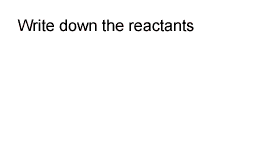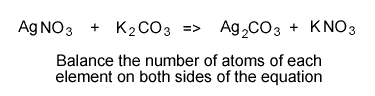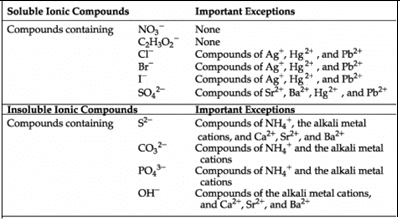Precipitation reactions and balanced equations This exercise will give you extra practice in writing ionic formulae and balanced chemical equations. As shown earlier we can identify the precipitate formed when two solutions are mixed together. For this unit of work you will need to be familiar with ionic formulae and chemical equations. Click to refresh yourself with precipitation reactions. Click to refresh yourself with ionic formulae Click to refresh yourself with chemical equations Example. A solution of silver nitrate and potassium carbonate are mixed. What products are formed ? Write the overall equation for this reaction. Step 1- Identify the products of this precipitation reaction by swapping the positive ions of the reactant compounds as shown on the right.Step 2 - Write the chemical equation with the reactants on the left and the products on the right. silver nitrate + potassium carbonate => silver carbonate + potassium nitrate. Step 3 - Write the correct formula for each reactant and product in the equation. View the chart of common ions if you need to. AgNO3 + K2CO3 => Ag2CO3 + KNO3 Step 4 - Balance the equation as shown in the animation below.Step 5 - Identify the precipitate and write the states of each product and reactant. States are written as subscripts. Solids have the subscript(s), while solutions are identified with (aq). AgNO3(aq) + K2CO3(aq) => Ag2CO3(s) + KNO3(aq) * Hint. Any compound that has the ammonium, potassium, sodium and or nitrate ions is always soluble and can never be a precipitate. Try the following exercises. You may view the chart of common ions in order to help you write the correct formula for each compound and use the solubility table (1) below, Write the balanced equation, include states, for the reaction between solutions of: a) potassium hydroxide and lead(II) nitrate (Click for the solution to a) b) lead(II) nitrate and iron(II) sulfate c) ammonium sulfate and barium chloride d) copper(II) sulfate and sodium carbonate e) silver nitrate and sodium chloride f) potassium hydroxide and copper(II) nitrate g) sodium hydroxide and iron(III) nitrate (Click for the solution to g) h) aluminium chloride and sodium hydroxide i) calcium nitrate and sodium carbonate j) copper(II) acetate and potassium sulfide k) lead(II) nitrate and potassium iodide l) magnesium sulfate and sodium hydroxide m) Barium hydroxide and copper(II) sulfate n) Calcium sulfide and aluminium sulfate Solutions If you found these difficult and you would like some extra exercises click hereSolubility table 1 Continue